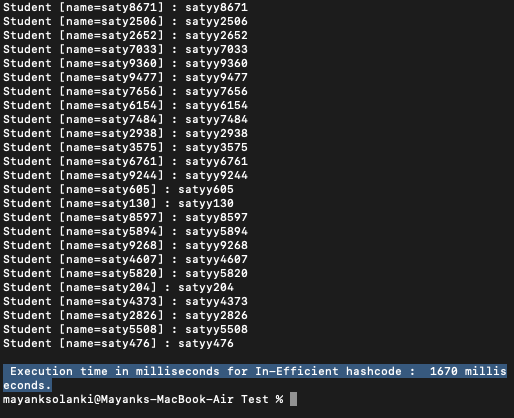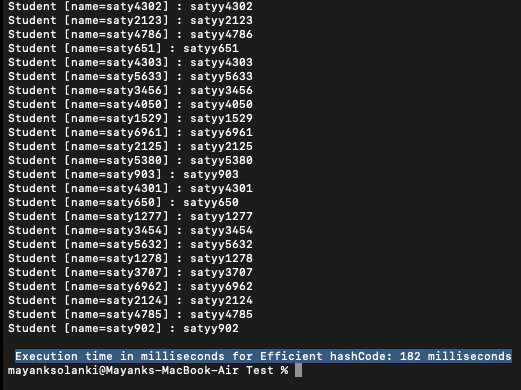GeeksforGeeks App
Open AppBrowser
Continue

# How Java 8 Helps in Improving Performance of HashMap?

Here we will be discussing out how we can, we improve the performance while using HashMap in Java, the Importance of the hashCode() contract and why is it very important to have an efficient hashcode, and what happens when we use an in-efficient hashcode. Let us directly roll over to implementing the same over the same set size of keys in our HashMap. It is as follows:

Implementation: Here no such concept is introduced, so a naive approach is being applied over our HashMap.

Example 1:

## Java

 `// Java Program to Illustrate In-efficient Technique``// While using HashMap`` ` `// Importing Map and HashMap utility classes``// from java.util package``import` `java.util.HashMap;``import` `java.util.Map;`` ` `// Main class``class` `HashMapEx2 {`` ` `    ``// main driver method``    ``public` `static` `void` `main(String[] args)``    ``{``        ``// Creating a Map object``        ``// Declaring object of user-defined class and string``        ``// type``        ``Map studentMap = ``new` `HashMap<>();``        ``long` `startTime = System.currentTimeMillis();``        ``for` `(``int` `i = ``0``; i < ``10000``; i++) {``            ``studentMap.put(``new` `Student(``"saty"` `+ i),``                           ``"satyy"` `+ i);``        ``}``        ``studentMap.forEach(``            ``(k, v) -> System.out.println(k + ``" : "` `+ v));``        ``long` `endTime = System.currentTimeMillis();``        ``long` `timeElapsed = endTime - startTime;``        ``System.out.println(``            ``"\n Execution time in milliseconds for In-Efficient hashcode :  "``            ``+ timeElapsed + ``" milliseconds."``);``    ``}``}`` ` `/*Student class.*/``class` `Student {``    ``String name;`` ` `    ``public` `Student(String name)``    ``{``        ``super``();``        ``this``.name = name;``    ``}`` ` `    ``@Override` `public` `String toString()``    ``{``        ``return` `"Student [name="` `+ name + ``"]"``;``    ``}`` ` `    ``/* Very in-efficient hashcode override */``    ``@Override` `public` `int` `hashCode()``    ``{``        ``return` `12``; ``/* Very inefficient hashcode, returns 12``                      ``for every key, that means all the keys ``                      ``will end up in the same bucket */``    ``}`` ` `    ``@Override` `public` `boolean` `equals(Object obj)``    ``{``        ``if` `(``this` `== obj)``            ``return` `true``;``        ``if` `(obj == ``null``)``            ``return` `false``;``        ``if` `(getClass() != obj.getClass())``            ``return` `false``;``        ``Student other = (Student)obj;``        ``if` `(name == ``null``) {``            ``if` `(other.name != ``null``)``                ``return` `false``;``        ``}``        ``else` `if` `(!name.equals(other.name))``            ``return` `false``;``        ``return` `true``;``    ``}``}`

Output: It contains humongous lines as the output to illustrate milliseconds taken hence, the last snapshot off the output roll is appended below in order to figure out the time taken for all the operations. It is as follows:Output Explanation: In the above program, we use Student as a HashMap key and the hashCode() override in the Student class is written very inefficiently which returns the same hashcode for every Student object.

Why an efficient hashcode is so darn important?

When you run the above program, what actually happens behind the scene is that when the HashMap is created it stores all the 10000 keys of student objects into the same bucket. As a result, all the keys get stored on a single bucket which results in a huge performance hit, and you can see that the time taken for the execution of the first program is approx 1700 milliseconds.

Now, in the program below, our hashcode override in the Student class is efficient and returns unique hashcode for every Student Object. As a result, each hashmap key is stored in a separate bucket, which improves the performance by ‘n’ times for storing the keys, and you can see that the time taken for the execution of the second program is only about 300 milliseconds

Example 2:

## Java

 `// Java Program to Illustrate In-efficient Technique``// While using HashMap`` ` `// Importing Map and HashMap utility classes``// from java.util package``import` `java.util.HashMap;``import` `java.util.Map;`` ` `// Main class``class` `HashMapEx3 {`` ` `    ``// main driver method``    ``public` `static` `void` `main(String[] args)``    ``{``        ``Map studentMap = ``new` `HashMap<>();``        ``long` `startTime = System.currentTimeMillis();``        ``for` `(``int` `i = ``0``; i < ``10000``; i++) {``            ``studentMap.put(``new` `Student(``"saty"` `+ i),``                           ``"satyy"` `+ i);``        ``}``        ``studentMap.forEach(``            ``(k, v) -> System.out.println(k + ``" : "` `+ v));``        ``long` `endTime = System.currentTimeMillis();``        ``long` `timeElapsed = endTime - startTime;``        ``System.out.println(``            ``"\n Execution time in milliseconds for Efficient hashCode: "``            ``+ timeElapsed + ``" milliseconds"``);``    ``}``}`` ` `/*Student class.*/``class` `Student {``    ``String name;``    ``public` `Student(String name)``    ``{``        ``super``();``        ``this``.name = name;``    ``}`` ` `    ``@Override` `public` `String toString()``    ``{``        ``return` `"Student [name="` `+ name + ``"]"``;``    ``}`` ` `    ``/* Efficient hashcode override */``    ``@Override` `public` `int` `hashCode()``    ``{``        ``return` `name.hashCode()``            ``* ``12``; ``/* Efficient hashcode, returns unique``                ``hashcode for every key, that``                ``means the keys will be distributed into``                ``unique buckets */``    ``}`` ` `    ``@Override` `public` `boolean` `equals(Object obj)``    ``{``        ``if` `(``this` `== obj)``            ``return` `true``;``        ``if` `(obj == ``null``)``            ``return` `false``;``        ``if` `(getClass() != obj.getClass())``            ``return` `false``;``        ``Student other = (Student)obj;``        ``if` `(name == ``null``) {``            ``if` `(other.name != ``null``)``                ``return` `false``;``        ``}``        ``else` `if` `(!name.equals(other.name))``            ``return` `false``;``        ``return` `true``;``    ``}``}`

Output: It contains humongous lines as the output to illustrate milliseconds taken hence, last snapshot off the output roll is appended below in order to figure out the time taken for all the operations. It is as follows:We can figure out a significant change wherein example 1 takes close to 1700 milliseconds and here as depicted from the output, it takes close to 180 seconds only. Hence, the multiplier of 10X is justified when we compare the above-demonstrated examples.

My Personal Notes arrow_drop_up
Related Tutorials